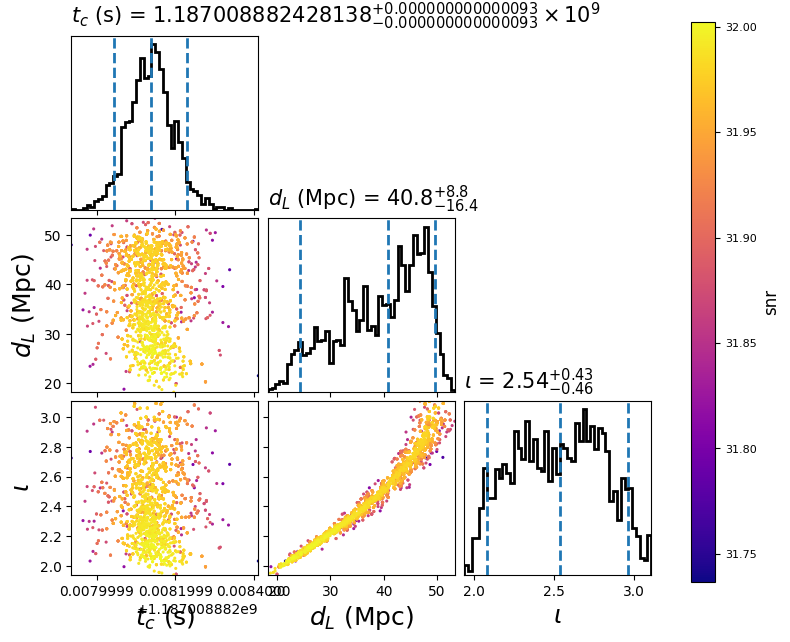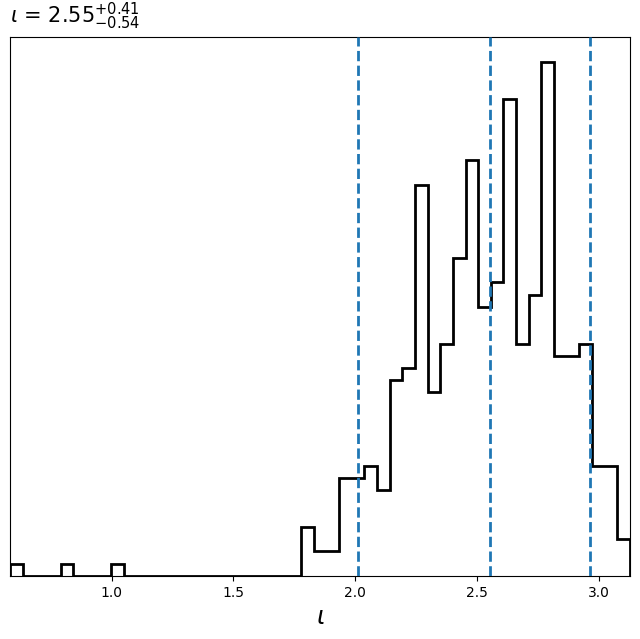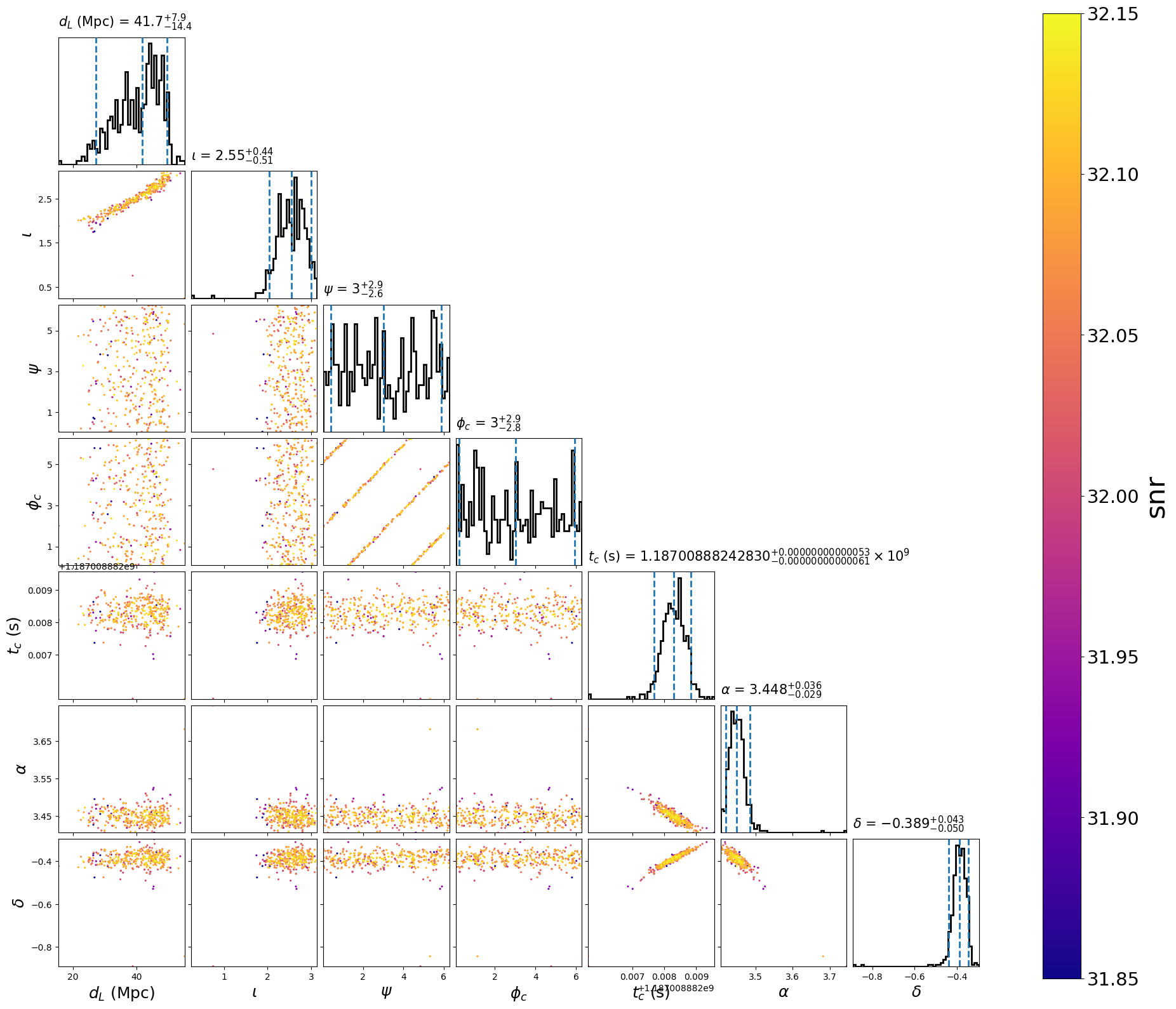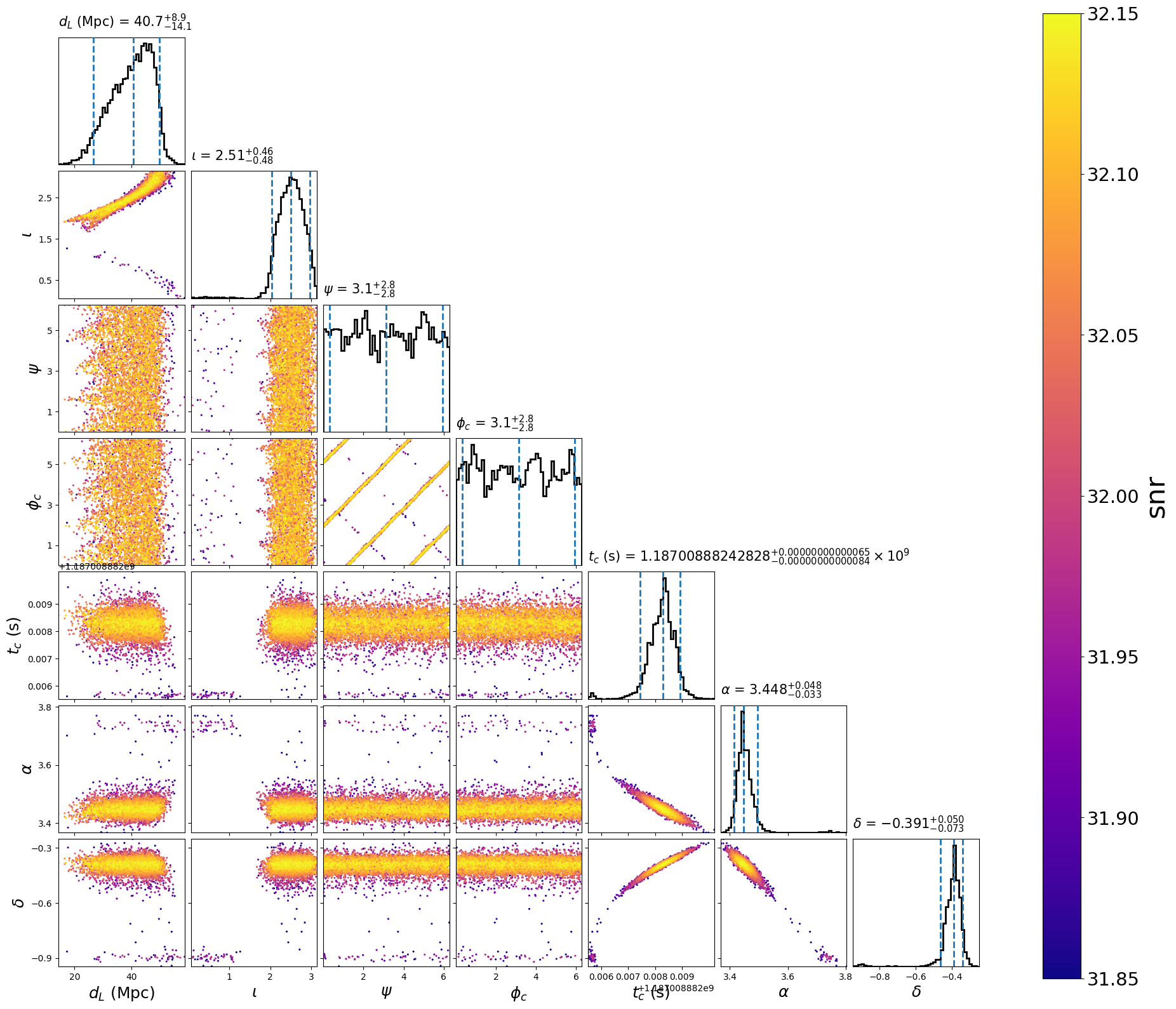# Using the single template model¶

## Quickstart example¶

The single template model is useful for when you know all the intrinsic parameters of your signal (say the masses, spins, etc of a merger). In this case, we don’t need to recalculate the waveform model to sample different possible extrinsic parameters (i.e. distance, sky location, inclination). This can greatly speed up the calculation of the likelihood. To use this model you provide the intrinsic parameters as fixed arguments as in the configuration file below.

This example demonstrates using the `single_template` model with the `dynesty` sampler. First, we create the following configuration file:

Configuration File
```[model]
name = single_template

#; This model precalculates the SNR time series at a fixed rate.
#; If you need a higher time resolution, this may be increased
sample_rate = 32768
low-frequency-cutoff = 30.0

[data]
instruments = H1 L1 V1
analysis-start-time = 1187008482
analysis-end-time = 1187008892
psd-estimation = median
psd-segment-length = 16
psd-segment-stride = 8
psd-inverse-length = 16
channel-name = H1:LOSC-STRAIN L1:LOSC-STRAIN V1:LOSC-STRAIN
frame-files = H1:H-H1_LOSC_CLN_4_V1-1187007040-2048.gwf L1:L-L1_LOSC_CLN_4_V1-1187007040-2048.gwf V1:V-V1_LOSC_CLN_4_V1-1187007040-2048.gwf
strain-high-pass = 15
sample-rate = 2048

[sampler]
name = dynesty
sample = rwalk
bound = multi
dlogz = 0.1
nlive = 200
checkpoint_time_interval = 100
maxcall = 10000

[variable_params]
; waveform parameters that will vary in MCMC
tc =
distance =
inclination =

[static_params]
; waveform parameters that will not change in MCMC
approximant = TaylorF2
f_lower = 30
mass1 = 1.3757
mass2 = 1.3757

#; we'll choose not to sample over these, but you could
polarization = 0
ra = 3.44615914
dec = -0.40808407

#; You could also set additional parameters if your waveform model supports / requires it.
; spin1z = 0

[prior-tc]
; coalescence time prior
name = uniform
min-tc = 1187008882.4
max-tc = 1187008882.5

[prior-distance]
#; following gives a uniform in volume
min-distance = 10
max-distance = 60

[prior-inclination]
name = sin_angle
```

`Download`

```set -e

for ifo in H-H1 L-L1 V-V1
do
file=\${ifo}_LOSC_CLN_4_V1-1187007040-2048.gwf
test -f \${file} && continue
curl -O --show-error --silent https://dcc.ligo.org/public/0146/P1700349/001/\${file}
done
```

By setting the model name to `single_template` we are using `SingleTemplate`.

Now run:

```pycbc_inference \
--config-file `dirname "\$0"`/single_simple.ini \
--nprocesses=1 \
--output-file single.hdf \
--seed 0 \
--force \
--verbose
```

`Download`

This will run the `dynesty` sampler. When it is done, you will have a file called `single.hdf` which contains the results. It should take about a minute or two to run.

To plot the posterior distribution, run:

```pycbc_inference_plot_posterior \
--input-file single.hdf \
--output-file single.png \
--z-arg snr
```

`Download`

This will create the following plot:The scatter points show position of different posterior samples. The points are colored by the log likelihood at that point, with the 50th and 90th percentile contours drawn.

## Marginalization subset of parameters¶

The single template model supports marginalization over its parameters. This can greatly speed up parameter estimation. The marginalized parameters can also be recovered as in the example below which extends the previous example to include all the parameters the model supports.

In this example, the sampler will only explore inclination, whereas all other parameters are either numerically or analytically marginalized over. Note that the marginalization still takes into account the priors you choose. This includes if you have placed boundaries or constraints.

First, you’ll need the configuration file.

Configuration File
```[model]
name = single_template

#; This model precalculates the SNR time series at a fixed rate.
#; If you need a higher time resolution, this may be increased
sample_rate = 8192
low-frequency-cutoff = 30.0

marginalize_vector_params = tc, ra, dec, polarization
marginalize_vector_samples = 1000

# These lines enable the marginalization to only use information
# around SNR peaks for drawing possible sky / time positions. Remove
# if you want to use the entire prior range as possible
peak_lock_snr = 4.0
peak_min_snr = 4.0

marginalize_phase = True

marginalize_distance = True
marginalize_distance_param = distance
marginalize_distance_interpolator = True
marginalize_distance_snr_range = 5, 50
marginalize_distance_density = 200, 200
marginalize_distance_samples = 1000

[data]
instruments = H1 L1 V1
analysis-start-time = 1187008482
analysis-end-time = 1187008892
psd-estimation = median
psd-segment-length = 16
psd-segment-stride = 8
psd-inverse-length = 16
channel-name = H1:LOSC-STRAIN L1:LOSC-STRAIN V1:LOSC-STRAIN
frame-files = H1:H-H1_LOSC_CLN_4_V1-1187007040-2048.gwf L1:L-L1_LOSC_CLN_4_V1-1187007040-2048.gwf V1:V-V1_LOSC_CLN_4_V1-1187007040-2048.gwf
strain-high-pass = 15
sample-rate = 2048

[sampler]
name = dynesty
dlogz = 0.5
nlive = 100

[variable_params]
; waveform parameters that will vary in MCMC
#coa_phase =
distance =
polarization =
inclination =
ra =
dec =
tc =

[static_params]
; waveform parameters that will not change in MCMC
approximant = TaylorF2
f_lower = 30
mass1 = 1.3757
mass2 = 1.3757

#; we'll choose not to sample over these, but you could
#polarization = 0
#ra = 3.44615914
#dec = -0.40808407

#tc = 1187008882.42825

#; You could also set additional parameters if your waveform model supports / requires it.
; spin1z = 0

#[prior-coa_phase]
#name = uniform_angle

#[prior-ra+dec]
#name = uniform_sky

[prior-ra]
name = uniform_angle

[prior-dec]
name = cos_angle

[prior-tc]
#; coalescence time prior
name = uniform
min-tc = 1187008882.4
max-tc = 1187008882.5

[prior-distance]
#; following gives a uniform in volume
min-distance = 10
max-distance = 60

[prior-polarization]
name = uniform_angle

[prior-inclination]
name = sin_angle
```

`Download`

Run this script to use this configuration file:

```pycbc_inference \
--config-file `dirname "\$0"`/single.ini \
--nprocesses=1 \
--output-file single_marg.hdf \
--seed 0 \
--force \
--verbose

pycbc_inference_plot_posterior \
--input-file single_marg.hdf \
--output-file single_marg.png \
--z-arg snr --vmin 31.85 --vmax 32.15

# This reconstructs any marginalized parameters
# and would be optional if you don't need them or
# have sampled over all parameters directly (see single.ini)
pycbc_inference_model_stats \
--input-file single_marg.hdf \
--output-file single_demarg.hdf \
--nprocesses 1 \
--reconstruct-parameters \
--force \
--verbose

pycbc_inference_plot_posterior \
--input-file single_demarg.hdf \
--output-file single_demarg.png \
--parameters distance inclination polarization coa_phase tc ra dec \
--z-arg snr --vmin 31.85 --vmax 32.15 \
```

`Download`

This will create the following plots:

Before demarginalization:After demarginalization:## Marginalization over all parameters¶

All parameters that the single_template model supports can be marginalized over to do so, you would just configure the model section to include each parameter (minus any you choose to be static). PyCBC Inference will simply reconstruct the missing parameters rather than performing any sampling over the parameter space. The number of samples to take from the posterior is configurable.

First, you’ll need the configuration file. Note that in the sampler section you can set the number of samples to draw from the posterior.

Configuration File
```[model]
name = single_template

#; This model precalculates the SNR time series at a fixed rate.
#; If you need a higher time resolution, this may be increased
sample_rate = 16384
low-frequency-cutoff = 30.0

marginalize_vector_params = tc, ra, dec, polarization, inclination
marginalize_vector_samples = 1000

marginalize_phase = True

marginalize_distance = True
marginalize_distance_param = distance
marginalize_distance_interpolator = True
marginalize_distance_snr_range = 5, 50
marginalize_distance_density = 200, 200
marginalize_distance_samples = 1000

[data]
instruments = H1 L1 V1
analysis-start-time = 1187008482
analysis-end-time = 1187008892
psd-estimation = median
psd-segment-length = 16
psd-segment-stride = 8
psd-inverse-length = 16
channel-name = H1:LOSC-STRAIN L1:LOSC-STRAIN V1:LOSC-STRAIN
frame-files = H1:H-H1_LOSC_CLN_4_V1-1187007040-2048.gwf L1:L-L1_LOSC_CLN_4_V1-1187007040-2048.gwf V1:V-V1_LOSC_CLN_4_V1-1187007040-2048.gwf
strain-high-pass = 15
sample-rate = 2048

[sampler]
name = dummy
num_samples = 5000

[variable_params]
; waveform parameters that will vary in MCMC
#coa_phase =
distance =
polarization =
inclination =
ra =
dec =
tc =

[static_params]
; waveform parameters that will not change in MCMC
approximant = TaylorF2
f_lower = 30
mass1 = 1.3757
mass2 = 1.3757

#; we'll choose not to sample over these, but you could
#polarization = 0
#ra = 3.44615914
#dec = -0.40808407

#tc = 1187008882.42825

#; You could also set additional parameters if your waveform model supports / requires it.
; spin1z = 0

#[prior-coa_phase]
#name = uniform_angle

#[prior-ra+dec]
#name = uniform_sky

[prior-ra]
name = uniform_angle

[prior-dec]
name = cos_angle

[prior-tc]
#; coalescence time prior
name = uniform
min-tc = 1187008882.4
max-tc = 1187008882.5

[prior-distance]
#; following gives a uniform in volume
min-distance = 10
max-distance = 60

[prior-polarization]
name = uniform_angle

[prior-inclination]
name = sin_angle
```

`Download`

Run this script to use this configuration file:

```pycbc_inference \
--config-file `dirname "\$0"`/single_instant.ini \
--nprocesses=1 \
--output-file single_instant.hdf \
--seed 0 \
--force \
--verbose

pycbc_inference_plot_posterior \
--input-file single_instant.hdf \
--output-file single_instant.png \
--parameters distance inclination polarization coa_phase tc ra dec \
--z-arg snr --vmin 31.85 --vmax 32.15 \
```

`Download`

This will create the following plot:

After demarginalization:## Abitrary sampling coordinates with nested samplers¶

The single template model also supports marginalization over the polarization angle by numerical sampling. The following example features arbitrary sampling coordinates with nested samplers using the `fixed_samples` distribution. Here we sample in the time delay space rather than sky location directly. This functionality is generic to pycbc inference and could be used with other models or samplers.

Configuration File
```[model]
name = single_template

#; This model precalculates the SNR time series at a fixed rate.
#; If you need a higher time resolution, this may be increased
sample_rate = 32768
low-frequency-cutoff = 30.0
marginalized_vector_samples = 100
marginalized_vector_params = polarization

[data]
instruments = H1 L1 V1
analysis-start-time = 1187008482
analysis-end-time = 1187008892
psd-estimation = median
psd-segment-length = 16
psd-segment-stride = 8
psd-inverse-length = 16
channel-name = H1:LOSC-STRAIN L1:LOSC-STRAIN V1:LOSC-STRAIN
frame-files = H1:H-H1_LOSC_CLN_4_V1-1187007040-2048.gwf L1:L-L1_LOSC_CLN_4_V1-1187007040-2048.gwf V1:V-V1_LOSC_CLN_4_V1-1187007040-2048.gwf
strain-high-pass = 15
sample-rate = 2048

[sampler]
name = dynesty
nlive = 100

[variable_params]
; waveform parameters that will vary in MCMC
tc =
distance =
inclination =
dh =
dhl =

[static_params]
; waveform parameters that will not change in MCMC
approximant = TaylorF2
f_lower = 30
mass1 = 1.3757
mass2 = 1.3757
#polarization = 0

[prior-tc]
; coalescence time prior
name = uniform
min-tc = 1187008882.4
max-tc = 1187008882.5

[prior-distance]
#; following gives a uniform in volume
min-distance = 10
max-distance = 60

[prior-inclination]
name = sin_angle

[prior-dh+dhl]
name = fixed_samples
subname = mysky
sample-size = 1e6

[mysky_sample-ra+dec]
name = uniform_sky

[mysky_transform-dh+dhl]
name = custom
inputs = ra, dec
dh = det_tc('H1', ra, dec, 1187008882.0, relative=1)
dhl = det_tc('L1', ra, dec, 1187008882.0, relative=1) - det_tc('H1', ra, dec, 1187008882.0, relative=1)

[waveform_transforms-ra+dec]
name = mysky
```